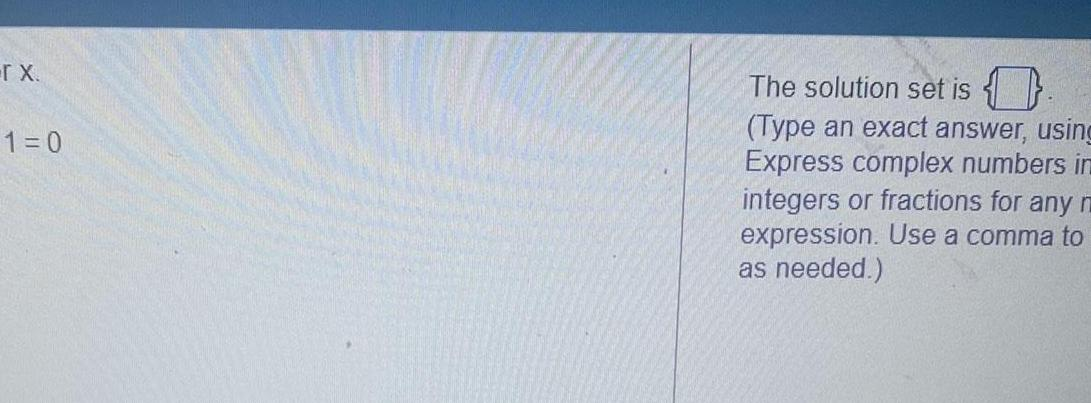Question:

# r X 1 0 The solution set is 0 Type an exact answer using

Last updated: 9/19/2023r X 1 0 The solution set is 0 Type an exact answer using Express complex numbers in integers or fractions for any r expression Use a comma to as needed